Courses

# NCERT Solutions - Open Economy Macroeconomics Commerce Notes | EduRev

## UPSC : NCERT Solutions - Open Economy Macroeconomics Commerce Notes | EduRev

The document NCERT Solutions - Open Economy Macroeconomics Commerce Notes | EduRev is a part of the UPSC Course NCERT Hindi Textbooks (Class 6 to Class 12).
All you need of UPSC at this link: UPSC

Page No 96:

Question 1: Differentiate between balance of trade and current account balance.
Answer: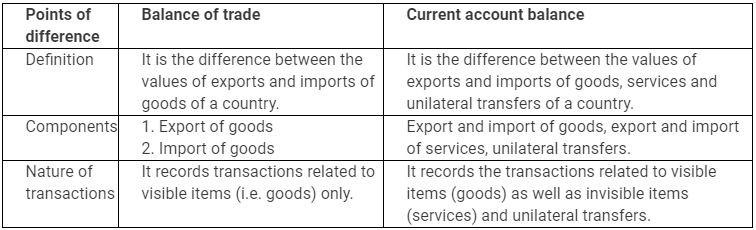Question 2: What are official reserve transactions? Explain their importance in the balance of payments.
Answer:
The transactions carried by monetary authority of a country, which cause changes in official reserves, are termed as official reserve transactions (ORT). These transactions are carried through purchase or sale of currency in the exchange market for foreign currencies or other assets. The reserves are drawn by selling foreign currencies in exchange market during deficits and foreign currencies are purchased during surplus. When the official reserves increases or decreases, it is called overall balance of payments surplus or deficit respectively.
Importance of ORT in balance of payments:
1. Purchase of a country’s own currency is a credit item in the balance of payments; whereas, sale of the currency is a debit item.
2. It helps to adjust the deficit and surplus in balance of payments.

Question 3: Distinguish between the nominal exchange rate and the real exchange rate. If you were to decide whether to buy domestic goods or foreign goods, which rate would be more relevant? Explain.
Answer: Nominal exchange rate is the price of one currency in terms of another. It is the amount of domestic currency required to buy one unit of foreign currency. For example a rupee-dollar exchange rate of Rs 45 means that it costs 45 rupees to buy 1 dollar.
Real exchange rate is the ratio of foreign prices to domestic prices. In other words, it measures foreign prices relative to domestic prices.
Real exchange rate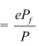Where Pf − price level of foreign currency
P − Price level of domestic currency
e − Nominal exchange rate
For example, if a watch costs \$40 in US and the nominal exchange rate is Rs 50 per US dollar, then, with real exchange rate of 1, it should cost Rs 2,000 (ePf = 50 × 40 = Rs 2000) in India.
If, I were to decide whether to buy domestic goods or foreign goods, then real exchange rate will be more relevant, because real exchange rate takes the inflation differential among the countries into account and is also used as an indicator of a country’s competitiveness in the foreign trade.

Question 4: Suppose it takes 1.25 yen to buy a rupee, and the price level in Japan is 3 and the price level in India is 1.2. Calculate the real exchange rate between India and Japan (the price of Japanese goods in terms of Indian goods). (Hint: First find out the nominal exchange rate as a price of yen in rupees).
Answer:
Price level in foreign country: (Japan) Pf = 3
Price level in home country: (India) P = 1.2
Now, real exchange rate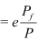Price of 1.25 yen = 1 rupee
Price of 1 yen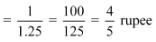Therefore,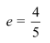So, real exchange rate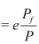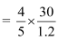= 2
Therefore, the real exchange rate is 2.

Question 5: Explain the automatic mechanism by which BoP equilibrium was achieved under the gold standard.
Answer:
Under the gold standard system, gold was taken as a common unit for measuring other country’s currency. Thus, the value of a currency was defined in terms of gold. The exchange rate in an open market was determined by its worth in terms of gold. It was fixed in lower limits and upper limits, under which it was allowed to fluctuate. So, the exchange rate became stable under gold standard. All the countries maintained stock of gold to exchange currency.

Question 6: How is the exchange rate determined under a flexible exchange rate regime?
Answer:
Under flexible exchange rate regime, the rate of exchange is determined by the forces of demand and supply. In other words, the equilibrium rate of exchange occurs where demand and supply are equal to each other. This can be illustrated with the help of the given figure: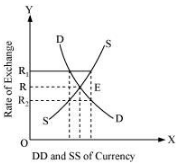In the figure, x−axis represents demand for and supply of foreign currency and y−axis represents the exchange rate. DD is the demand curve that is downward slopping, showing an inverse relationship between the rate of exchange and demand for foreign currency. Whereas, the supply curve is upward sloping, showing positive relationship between the rate of exchange and the supply of foreign currency. E is the equilibrium rate of exchange, where the demand equates the supply of foreign exchange (OR). Now, if the exchange rate rises to OR1, then the supply exceeds the demand, forcing the exchange rate to fall back to OR. On the contrary, if the exchange rate falls to OR2, there is excess demand over supply. Hence, the rate of exchange rises from R2 to R. Hence, the equilibrium exchange rate (OR) is determined by demand and supply of foreign currency.

Question 7: Differentiate between devaluation and depreciation.
Answer: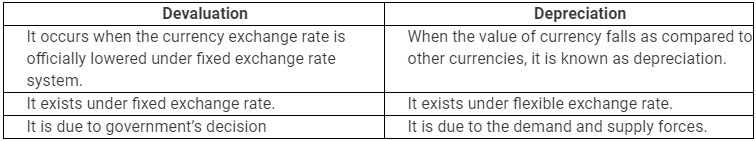Question 8:Would the central bank need to intervene in a managed floating system? Explain why.
Answer: Managed floating system is a combination of two systems − fixed and floating exchange rate systems. It calls for the government or central bank to intervene when the need for the same is needed. The government or the central bank helps in moderating the exchange rate movements by purchasing and selling of foreign currency. Thus, to avoid dirty floating, the government exercises its power to intervene, whenever the need arises.

Question 9: Are the concepts of demand for domestic goods and domestic demand for goods the same?
Answer: In a closed economy, the demand for domestic goods and domestic demand for goods are similar terms. However, in an open economy, these two terms have different meanings. Demand for domestic goods includes both domestic and foreign demand for domestic goods. Whereas, domestic demand for goods refers to the domestic market demand of a country, that is either produced domestically or abroad (foreign countries).

Question 10: What is the marginal propensity to import when M = 60 + 0.06Y? What is the relationship between the marginal propensity to import and the aggregate demand function?
Answer:
Marginal propensity to import is the fraction of additional income spent on imports.
It is given that M = 60 + 0.06Y
Therefore, marginal propensity to import (m) = 0.06. It reflects induced imports; that is the part of the total imports, which is a function of income.
Since the marginal propensity to import negatively affects the aggregate demand function, when income increases the aggregate demand decreases. This is because the additional income is spent on foreign goods and not on domestic products.

Question 11: Why is the open economy autonomous expenditure multiplier smaller than the closed economy one?
Answer: In case of a closed economy, equilibrium level of income is given by
Y = C + cY + I + G
Or, Y − cY = C + I + G
Or, Y (1 − c) = C + I + G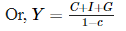Let, (C + I + G) = A1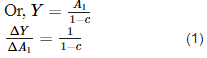In the case of an open economy, equilibrium level of income is given by
Y = C + cY + I + G + X − M − mY
Or, Y − cY + mY = C + I + G + X − M
Or, Y (1 − c + m) = C + I + G + X − M
Or,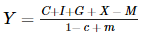Let autonomous expenditure (A2) = C + I + G + X − M
Or,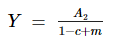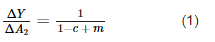Comparing equations (1) and (2) and the denominators of the two multipliers, we can conclude that multiplier in an open economy is smaller than that in a closed economy, as the denominator in an open economy is greater than denominator in a closed economy.

Question 12: Calculate the open economy multiplier with proportional taxes, T = tY, instead of lump−sum taxes as assumed in the text.
Answer:
In the case of proportional tax, the equilibrium income would be
Y = C + c (1 − t) Y + I + G + X − M − mY
Y − c (1 − t) Y + mY = C + I +G + X − M
Y[1 − c (1 − t) +m] = C + I + G + X − M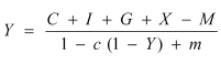Autonomous expenditure (A) = C + I + G + X − M
Therefore, open economy multiplier with proportional taxes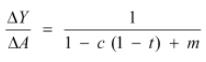Question 13: Suppose C = 40 + 0.8Y D. T = 50, I = 60, G = 40, X = 90, M = 50 + 0.05Y
(a) Find equilibrium income
(b) Find the net export balance at equilibrium income
(c) What happens to equilibrium income and the net export balance when the government purchases increase from 40 to 50?
Answer:

C = 40 + 0.8YD
T = 50
I = 60
G = 40
X = 90
M = 50 + 0.05Y
(a) Equilibrium level of income
Y = C + c (Y − T) + I + G + X − M ­− mY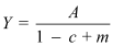Where, A = C − CT + I + G + X − M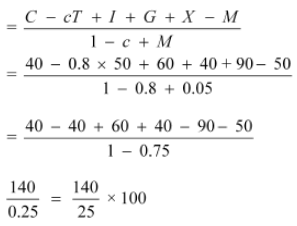= 560
(b) Net exports at equilibrium income
NX = X − M − mY
= 90 − 50 − 0.05 × 560
= 40 − 28 = 12
(c) When G increase from 40 to 50,
Equilibrium income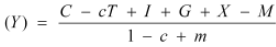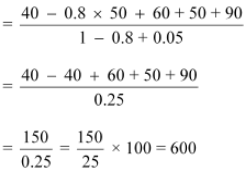Net export balance at equilibrium income
NX = X − (M − mY)
= 90 − 50 + 0.05 × 600
= 40 − 30 = 10

Question 14: In the above example, if exports change to X = 100, find the change in equilibrium income and the net export balance.
Answer:

C = 40 + 0.8 YD
T = 50
I = 60
G = 40
X = 100
M = 50 + 0.05Y
Equilibrium income (Y)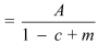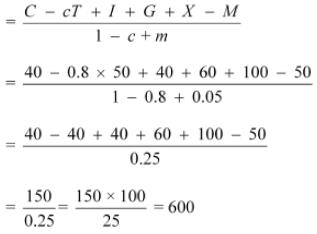Net export balance NX = X − M − 0.05Y
= 100 − 50 − 0.05 × 600
= 50 − 0.05 × 60
= 50 − 30 = 20

Question 15: Explain why G − T = (Sg − I) − (X − M).
Answer: In a closed economy, savings and investments are equal at equilibrium level of income. However, in an open economy savings and investments differ.
Y = C + I + G + X − M
or, Y = C + I + G + NX        [as NX = X − M]
or, Y − C − G = I + NX         ...(1)
Now, the component on the LHS can be regarded as national savings. That is, net national income which is left after consumption and government spending. Getting back to the equation (1), we can say:
S = I + NX
National savings (S) can be segregated into Private Savings (SP) and Government Savings (Sg).
so, SP + Sg = I + NX
or, NX = SP + Sg − I
or, NX = (Y − C − T) + (T − G) − I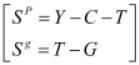or, NX = Y − C − T + T − G −I
or, NX = Y − C − G − I
or, G = Y − C − I − NX
or, G − T = Y − C − I − NX − T    [Subtracting T from both sides]
or, G − T = Y − C − T − I − NX
or, G − T = (SP − I) − NX
Note : There is a misprint in the question as the equation mentions Sg  instead of SP. The correct equation is G − T = (SP − I) − NX.

Question 16: If inflation is higher in country A than in Country B, and the exchange rate between the two countries is fixed, what is likely to happen to the trade balance between the two countries?
Answer: Country A has a higher inflation than country B. Since, the exchange rate is fixed, it is advantageous for country B to export goods to country A. Similarly, it is advantageous for country A to import goods from country B. On the other hand, it would be expensive for country A to export goods to country B. Thus, country A will have trade deficit as it will import more goods as compared to exports, from country B. Country B will import less goods as compared to exports, from country A. Hence, there is a trade surplus in country B.

Question 17: Should a current account deficit be a cause for alarm? Explain.
Answer:
Current account deficit is the excess of total imports of goods, services and transfers over total exports of goods, services and transfers. This situation makes a country debtor to the rest of the world. But, this cannot be always treated as a cause for alarm because countries might be running in deficits (current account) to increase productivity and exports in future. Also, more investment will help in building capital stock, which in future will lead to rise in output.

Question 18: Suppose C = 100 + 0.75Y D, I = 500, G = 750, taxes are 20 per cent of income, X = 150, M = 100 + 0.2Y. Calculate equilibrium income, the budget deficit or surplus and the trade deficit or surplus.
Answer:

C = 100 + 0.75YD
I = 500
G = 750
X = 150
M = 100 + 0.2Y
Or, Y = 100 + .75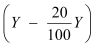+ 500 +750 + 150 − 100 − 0.2Y
Or,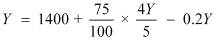Or,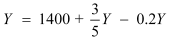Or,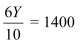Or,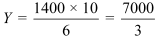Government expenditure = 750
Government receipts (taxes)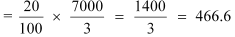Since, government expenditure > government receipts
It shows the government is running budget deficit
NX = X − M − MY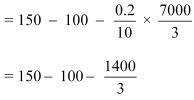= 150 − 100 − 466.66
= 150 − 566.66
= − 416.66
Since NX is negative, it implies trade deficit.

Question 19: Discuss some of the exchange rate arrangements that countries have entered into to bring about stability in their external accounts.
Answer:
To combine the two extreme positions, `fixed’ and ‘flexible’, the following exchange rate arrangements are used by governments to bring stability in external accounts:
1. Wider Bands
A system that allows adjustment in fixed exchange rate is referred to as wider bands. It permits only 10% variation between the currencies of any two countries. For example, a country can improve its balance of payments (BoP) deficit by depreciating it’s currency, which leads to increase in demand for domestic goods due to increase in purchasing power of other currencies. This further leads to the increase in exports, hence improving the BoP.
2. Crawling Peg
Crawling peg system allows continuous and regular adjustments in the exchange rate. Only 1% of variation is allowed at a time.
3. Managed floating
Managed floating is a scheme under which government can intervene to vary the exchange rate when the situation demands so. There is no specific limit of variation as in crawling peg and wider bands.

Offer running on EduRev: Apply code STAYHOME200 to get INR 200 off on our premium plan EduRev Infinity!

## NCERT Hindi Textbooks (Class 6 to Class 12)

974 docs|526 tests

,

,

,

,

,

,

,

,

,

,

,

,

,

,

,

,

,

,

,

,

,

;